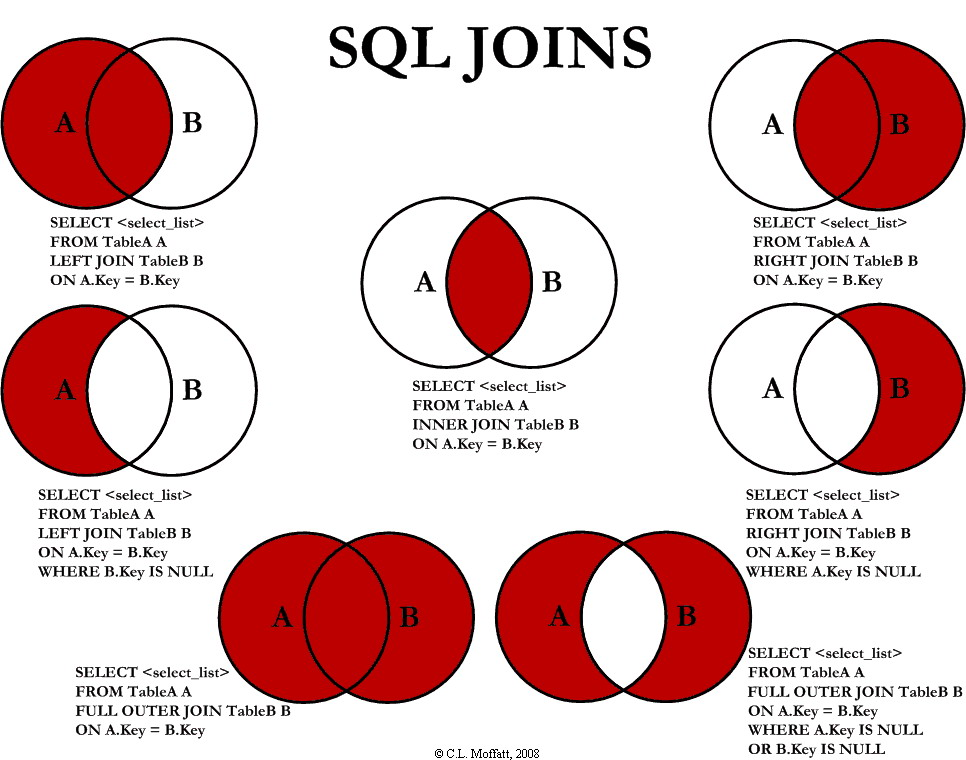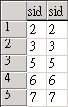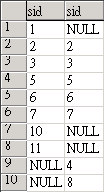## SQL Join語法圖示select a.sid from table_a a left join table_b b on a.sid=b.sid而 right join (也就是 right outer join), 則是取出以右邊為主, 不管左邊是否存在的資料, 如:

select a.sid from table_a a right join table_b b on a.sid=b.sidselect a.sid, b.sid from table_a a full join table_b b on a.sid=b.sid## 反向排序內容(倒排)

```//var arrData = document.getElementById("data").value.split("\n");
//arrData = arrData.reverse();
//document.getElementById("data").value = arrData.join("\n");
document.getElementById("data").value = document.getElementById("data").value.split("\n").reverse().join("\n");
```